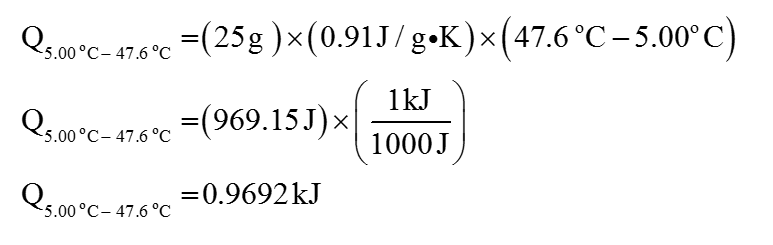# normal boiling point of C2Cl3F3 (MW=187.4) is 47.6oC. The specific heats for the liquid and gas are 0.91 J/gK and 0.67 J/gK, respectively. Calculate the heat required to convert 25.0 g of C2Cl3F3 from a liquid at 5.00oC to a gas at 82.00oC if ΔvapH = 27.49 kJ/mol.

Question
15 views

normal boiling point of C2Cl3F3 (MW=187.4) is 47.6oC. The specific heats for the liquid and gas are 0.91 J/gK and 0.67 J/gK, respectively. Calculate the heat required to convert 25.0 g of C2Cl3F3 from a liquid at 5.00oC to a gas at 82.00oC if ΔvapH = 27.49 kJ/mol.

check_circle

The heat has to be calculated.

Given,

Normal melting point of C2Cl3F3 is 47.6 oC. Specific heat for the liquid and gas are 0.91 J/gK and 0.67 J/Gk....

### Want to see the full answer?

See Solution

#### Want to see this answer and more?

Solutions are written by subject experts who are available 24/7. Questions are typically answered within 1 hour.*

See Solution
*Response times may vary by subject and question.
Tagged in

### Chemistry